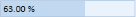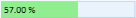# Range Adorner in WPF Percent TextBox

Value of PercentTextBox can be visually indicated like a progress bar using range-adorner feature, this feature is disabled by default. You can show the adorner over `PercentTextBox` control by setting EnableRangeAdorner property to `true`. default value of `EnableRangeAdorner` is `false`. The adorner layer can be filled in the control area on the basis of the minimum and maximum values with considering the given value. Range Adorner is not displayed when a `MinValue` or `MaxValue` property is not set.

``<syncfusion:PercentTextBox x:Name="percentTextBox" MinValue="0" PercentValue="63" MaxValue="100" EnableRangeAdorner="True" />``
``````PercentTextBox percentTextBox = new PercentTextBox();
percentTextBox.MinValue = 0;
percentTextBox.MaxValue = 100;
percentTextBox.PercentValue = 63;``<syncfusion:PercentTextBox x:Name="percentTextBox" MinValue="0" MaxValue="100" PercentValue="57" EnableRangeAdorner="True" RangeAdornerBackground="LightGreen"/>``
``````PercentTextBox percentTextBox = new PercentTextBox();